# The Grid Method of Multiplication

The Grid Method of Multiplication• The grid method is a way of multiplying two numbers with more than one .
• We can 24 into 20 + 4.
• We then partition 15 into 10 + 5.
• Write the partitioned parts of each number outside the rows and columns of the grid.
• Multiply the 2 numbers that are on the outside of each box and write the answer inside each box.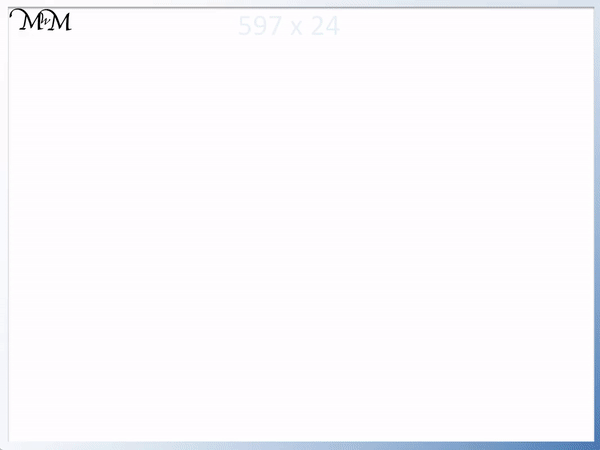• Having partitioned our numbers, we multiply each row and column together and write the answers in grid.
• 20 x 10 = 200.
• 20 x 5 = 100.
• 4 x 10 = 40
• 4 x 5 = 20
• Now that we have multiplied all of the partitioned values we add all the numbers inside the boxes together to get the final answer.
• In the top row: 200 + 100 = 300.
• In the second row: 40 + 20 = 60.
• The final answer is 300 + 60 = 360.#### The Grid Method of Multiplication (2-and-3-Digit Numbers)# The Grid Method of Multiplication

## What is the Grid Method of Multiplication?

The grid method is a written method of multiplication that is used to multiply two numbers that contain more than one digit. It involves partitioning the numbers into their place value columns and multiplying each part separately. These results are written in a grid and the final answer is found by adding them up to make a total.

Grid method multiplication may also be referred to as box method multiplication or grammar school multiplication.

Here is an example of the grid method used to multiply 254 × 63.

The two numbers are partitioned into 200 + 50 + 4 and 60 + 3.In the grid method, each box has two partitioned numbers next to it. One number is above the box and one number is to the left of the box.

We multiply these two numbers together and write the answer in each box of the grid.

2 x 6 = 12

So, 200 x 60 = 12 000

We write ‘12 000’ where their column and row meet.Next, we’ll multiply 200 by 3.

2 x 3 = 6

So, 200 x 3 = 600

We write ‘600’ in the corresponding box.Now, we’ll multiply 50 by 60.

5 x 6 = 30

So, 50 x 60 = 3000Now, we’ll multiply 50 by 3.

5 x 3 = 15

So, 50 x 3 = 150Now, we’ll multiply 4 by 60.

4 x 6 = 24

So, 4 x 60 = 240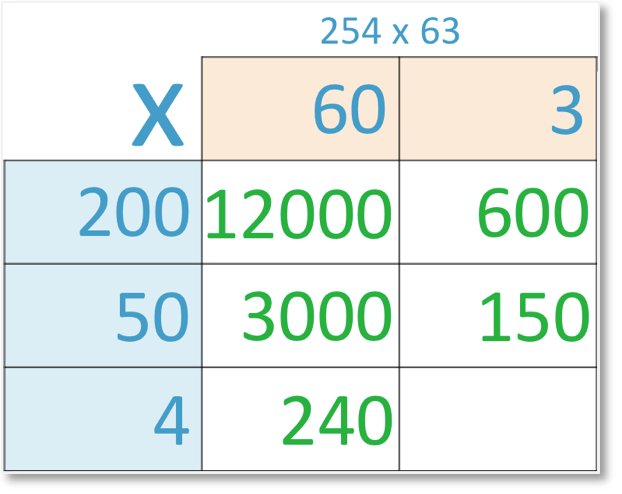Finally, we’ll multiply 4 by 3.

4 x 3 = 12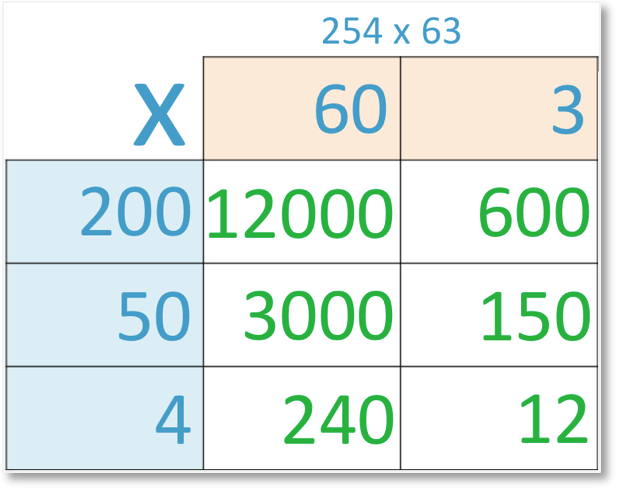Once all of the boxes in the grid are filled, these answers are added together to make a total.

We can add the numbers row by row and use column addition.

We’ll begin by adding the numbers in the first row of our grid.

12 000 + 600 = 12 600Next, we add the numbers in the middle row of our grid.

3000 + 150 = 3150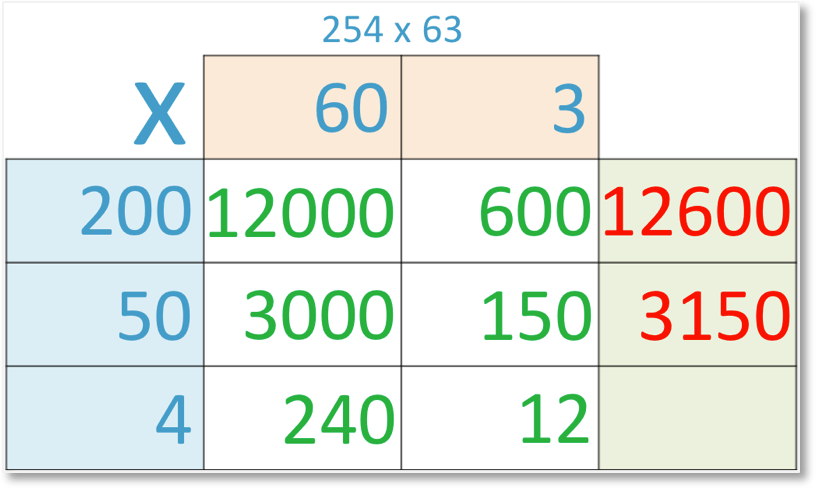Finally, we add the numbers in the bottom row of our grid.

240 + 12 = 252We can now use column addition to find the total of the row totals.

12 600 + 3150 + 252 = 16 002.Therefore 254 x 63 = 16002.

## Why Use the Grid Method?

The grid method is used to provide structure to multiplying larger numbers as an alternative to long multiplication. This structure generally helps students to avoid mistakes as it breaks the multiplication down further and is easier to understand.

Whilst the grid method can be used as an alternative to the long multiplication method, an understanding of grid method multiplication is worthwhile in itself as the same concepts of multiplication can be applied to other mathematical topics such as area and algebra.

## How to Do the Grid Method of Multiplication

To do the grid method of multiplication, follow these steps:

1. Draw a grid containing as many columns as the number of digits in the first number and as many rows as the number of digits in the second number.
2. Partition the first number into its place value columns and write each part above each of the boxes in the grid.
3. Partition the second number into its place value columns and write each part to the left of each of the boxes in the grid.
4. For each box multiply the number above it by the number to the left of it and write the answer inside the box.
5. Add up the total of the numbers in each of the boxes to find the final answer.

For example, here is the grid method of multiplying 24 x 15 step-by-step.

The first step is to draw a grid containing the same number of rows as the number of digits in the first number and the same number of columns as the number of digits in the second number.

Both of the numbers 24 and 63 have 2 digits and so, we draw a grid with 2 rows and 2 columns.

The second step is to partition each number into its place value columns and write these above and to the left of each box respectively.

24 = 20 + 4 and 63 = 60 + 3.The next step is to multiply the numbers above and to the left of each box and write the answer inside each box.

20 x 10 = 200Next, we’ll multiply 20 by 5.

20 x 5 = 100

We write ‘100’ in the corresponding box.Now, we’ll multiply 10 by 4.

10 x 4 = 40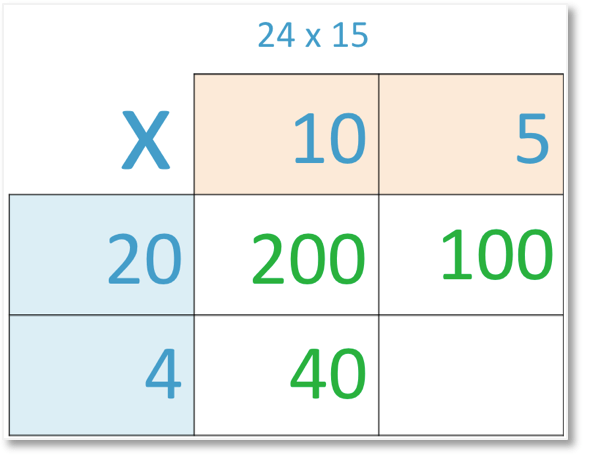Finally, we’ll multiply 4 by 5.

4 x 5 = 20The next step is to add the numbers in each box of the grid to find the total. We can add the numbers row by row to break this down.

We’ll start by adding the first row of our grid and writing the answer in the box at the end of this first row.

200 + 100 = 300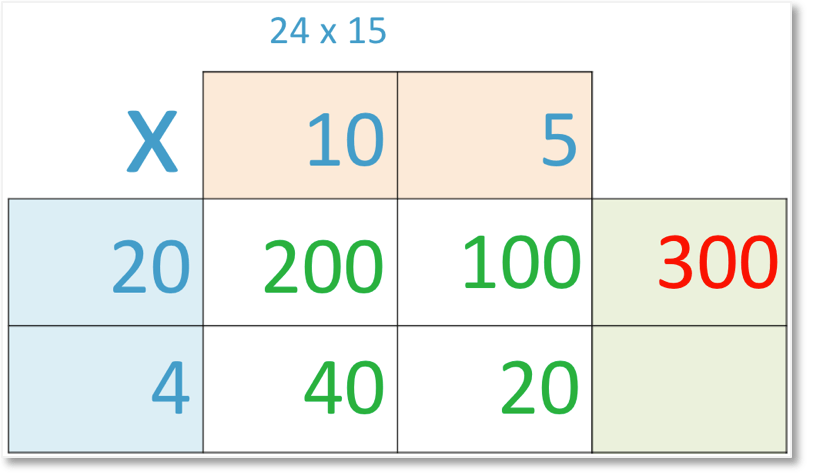Next, we’ll add the second row of our grid.

40 + 20 = 60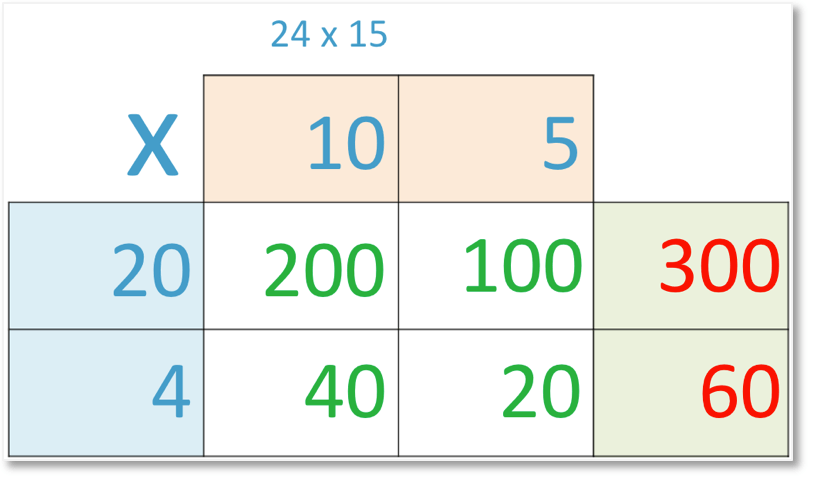The sum of the two row totals is 300 + 60 = 360.Therefore using grid method multiplication, 24 x 15 = 360.Now try our lesson on Lattice Multiplication where we learn how to use the lattice method of multiplication.

error: Content is protected !!